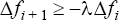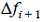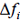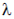# LINEAR_SOLVER_PARAMETERS

Specifies the parameters for fine tuning the linear equation solver.

AcuSolve Command

## Syntax

LINEAR_SOLVER_PARAMETERS {parameters}

## Qualifier

This command has no qualifier.

## Parameters

lhs_storage or storage (enumerated) [=reduced_memory_sparse]
Type of storage for the left-hand-side (LHS] matrices.
sparse
Compact sparse format.
reduced_memory_sparse or fast_sparse
Single-precision compact sparse format.
min_num_iteration_ratio (real) >=0 <=1 [=0.5]
Number of iterations must be at least this ratio times the previous number of iterations (of the same stagger).
pressure_precedence_factor (real) > = 0 [=1]
Relaxation factor to relax the pressure equation left-hand side (LHS) matrix relative to the continuity equation. This is an advanced option and is rarely changed from the default value.
num_pressure_projection_vectors or pres_projs
Number of available pressure projection vectors for pressure, flow, and temperature_flow staggers.
num_velocity_projection_vectors or vel_projs (integer) >=0 [=5]
Number of available velocity projection vectors for velocity, flow, and temperature_flow staggers.
num_flow_projection_vectors or flow_projs (integer) >=0 [=5]
Number of available flow projection vectors for flow and temperature_flow staggers.
num_temperature_projection_vectors or temp_projs (integer) >=0 [=10]
Number of available temperature projection vectors for temperature and temperature_flow staggers.
Number of available radiation projection vectors for temperature and temperature_flow staggers.
num_temperature_flow_projection_vectors or temp_flow_projs (integer) >=0 [=4]
Number of available temperature-flow projection vectors for the temperature_flow staggers.
num_species_projection_vectors or species_projs (integer) >=0 [=10]
Number of available species projection vectors for species staggers.
num_turbulence_projection_vectors or turb_projs (integer) >=0 [=10]
Number of available turbulence projection vectors for turbulence staggers.
num_mesh_displacement_projection_vectors or mesh_projs (integer) >=0 [=10]
Number of available mesh displacement projection vectors for mesh displacement staggers.
num_viscoelastic_stress_projection_vectors or vest_projs (integer) >=0 [=5]
Number of available viscoelastic stress projection vectors for viscoelastic_stress staggers.
num_field_projection_vectors or field_projs (integer) >=0 [=10]
Number of available field projection vectors for field staggers.
num_levelset_projection_vectors or ls_projs (integer) >=0 [=10]
Number of available levelset projection vectors for levelset staggers.
num_redistancing_projection_vectors or rdist_projs (integer) >=0 [=10]
Number of available redistancing projection vectors for redistancing staggers.
num_transition_projection_vectors or ttm_projs (integer) >=0 [=10]
Number of available turbulence transition projection vectors for transition staggers.
num_elec_potential_projection_vectors or elecp_projs (integer) >=0 [=10]
Number of available electric potential projection vectors for charge conservation staggers.
pressure_lhs_inverse_order (integer) >=0 [=5]
Polynomial order used to approximate the inverse of the pressure equation LHS for pressure projection.
velocity_lhs_inverse_order (integer) >=0 [=5]
Polynomial order used to approximate the inverse of the velocity equation LHS for velocity projection.
flow_lhs_inverse_order (integer) >=0 [=5]
Polynomial order used to approximate the inverse of the flow equation LHS for flow projection.
temperature_lhs_inverse_order (integer) >=0 [=5]
Polynomial order used to approximate the inverse of the temperature equation LHS for temperature projection.
topology_pressure_lhs_inverse_order (integer) >=0 [=5]
Polynomial order used to approximate the inverse of the topology pressure equation LHS for topology pressure projection.
topology_velocity_lhs_inverse_order (integer) >=0 [=5]
Polynomial order used to approximate the inverse of the topology velocity equation LHS for topology velocity projection.
topology_flow_lhs_inverse_order (integer) >=0 [=5]
Polynomial order used to approximate the inverse of the topology flow equation LHS for topology flow projection.
pressure_regularization_factor or pres_reg_fct (real) >=0 [=1.0]
Regularization factor used within the discrete pressure Poisson equation.
velocity_regularization_factor or vel_reg_fct (real) >=0 [=0.5]
Regularization factor used for the solution of the velocity equation.
flow_regularization_factor or flow_reg_fct (real) >=0 [=0]
Regularization factor used for the solution of the flow equation.
temperature_regularization_factor or temp_reg_fct (real) >=0 [=0]
Regularization factor used for the solution of the temperature equation.
temperature_flow_regularization_factor or temp_flow_reg_fct (real) >=0 [=0]
Regularization factor used for the solution of the coupled temperature-flow equation.
species_regularization_factor or species_reg_fct (real) >=0 [=0]
Regularization factor used for the solution of the species equation.
turbulence_regularization_factor or turb_reg_fct (real) >=0 [=0]
Regularization factor used for the solution of the turbulence equation.
num_topology_pressure_projection_vectors or topo_pres_projs (integer) >=0 [=10]
Number of available pressure projection vectors for pressure, flow, and temperature_flow staggers.
num_topology_velocity_projection_vectors or topo_vel_projs (integer) >=0 [=5]
Number of available velocity projection vectors for velocity, flow, and temperature_flow staggers.
num_topology_flow_projection_vectors or topo_flow_projs (integer) >=0 [=5]
Number of available flow projection vectors for flow and temperature_flow staggers.
mesh_displacement_regularization_factor or mesh_reg_fct (real) >=0 [=0]
Regularization factor used for the solution of the mesh displacement equation.
viscoelastic_stress_regulation_factor or vest_reg_fct (real) >=0 [=0]
Regularization factor used for the solution of the viscoelastic stress equation.
field_regularization_factor or field_reg_fct (real) >=0 [=0]
Regularization factor used for the solution of the field equation.
levelset_regularization_factor or ls_reg_fct (real) >=0 [=0]
Regularization factor used for the solution of the levelset equation.
redistancing_regularization_factor or rdist_reg_fct (real) >=0 [=0]
Regularization factor used for the solution of the redistancing equation.
transition_regularization_factor or ttm_reg_fct (real) >=0 [=0]
Regularization factor used for the solution of the turbulence transition equation.
elec_potential_regularization_factor or elecp_reg_fct (real) >=0 [=0]
Regularization factor used for the solution of the charge conservation equation.
pressure_update_factor or pres_upd_fct (real) >0 <=1 [=1.0]
Update factor used for the solution increment of pressure.
velocity_update_factor or vel_upd_fct (real) >0 <=1 [=1.0]
Update factor used for the solution increment of velocity.
temperature_update_factor or temp_upd_fct (real) >0 <=1 [=1.0]
Update factor used for the solution increment of temperature.
species_update_factor or species_upd_fct (real) >0 <=1 [=1.0]
Update factor used for the solution increment of species.
turbulence_update_factor or turb_upd_fct (real) >0 <=1 [=1.0]
Update factor used for the solution increment of turbulence.
topology_pressure_update_factor or topo_pres_upd_fct (real) >0 <=1 [=1.0]
Update factor used for the solution increment of topology pressure.
topology_velocity_update_factor or topo_vel_upd_fct (real) >0 <=1 [=1.0]
Update factor used for the solution increment of topology velocity.
mesh_displacement_update_factor or mesh_upd_fct (real) >0 <=1 [=1.0]
Update factor used for the solution increment of mesh displacement.
viscoelastic_stress_update_factor or vest_upd_fct (real) >0 <=1 [=1.0]
Update factor used for the solution increment of the viscoelastic stress.
Update factor used for the solution increment of radiation heat flux for enclosure radiation. For participating media radiation, this is the update factor for the solution increment of the incident radiation and the radiative intensity for the p1_model and discrete_ordinate radiation models, respectively.
field_update_factor or field_upd_fct (real) >0 <=1 [=1.0]
Update factor used for the solution increment of field.
levelset_update_factor or ls_upd_fct (real) >0 <=1 [=1.0]
Update factor used for the solution increment of levelset.
redistancing_update_factor or rdist_upd_fct (real) >0 <=1 [=1.0]
Update factor used for the solution increment of redistancing.
transition_update_factor or tts_upd_fct (real) >0 <=1 [=1.0]
Update factor used for the solution increment of turbulence transition.
elec_potential_update_factor or elecp_upd_fct (real) >0 <=1 [=1.0]
Update factor used for the solution increment of charge conservation equation.
max_pressure_update or max_pres_upd (real) >=0 [=0]
Maximum amount each pressure nodal value may be updated at each solution update of a stagger. If zero there is no limit.
max_velocity_update or max_vel_upd (real) >=0 [=0]
Maximum amount each velocity nodal value may be updated at each solution update of a stagger. If zero there is no limit.
max_temperature_update or max_temp_upd (real) >=0 [=0]
Maximum amount each temperature nodal value may be updated at each solution update of a stagger. If zero there is no limit.
max_species_update or max_species_upd (real) >=0 [=0]
Maximum amount each pressure nodal value may be updated at each solution update of a stagger. If zero there is no limit.
max_turbulence_update or max_turb_upd (real) >=0 [=0]
Maximum amount each turbulence nodal value may be updated at each solution update of a stagger. If zero there is no limit.
max_topology_pressure_update or max_topo_pres_upd (real) >=0 [=0]
Maximum amount each topology pressure nodal value may be updated at each solution update of a stagger. If zero there is no limit.
max_topology_velocity_update or max_topo_vel_upd (real) >=0 [=0]
Maximum amount each topology velocity nodal value may be updated at each solution update of a stagger. If zero there is no limit.
max_mesh_displacement_update or max_mesh_upd (real) >=0 [=0]
Maximum amount each pressure nodal value may be updated at each solution update of a stagger. If zero there is no limit.
max_viscoelastic_stress_update or max_vest_upd (real) >=0 [=0]
Maximum amount each viscoelastic stress nodal value may be updated at each solution update of a stagger. If zero there is no limit.
Maximum amount each radiation heat flux nodal value may be updated at each solution update of a stagger of an enclosure radiation stagger. For radiation with a participating medium, this is the maximum amount each nodal values of incident radiation or radiation intensity (for p1_model and discrete_ordinate radiation models, respectively) may be updated at each solution update of a p1_model or discrete_ordinate radiation stagger. If zero there is no limit.
max_reverse_update_factor (real) >=0 [=0]
Maximum negative amount each nodal solution increment may change as a fraction of the previous solution increment. If zero there is no limit.
max_field_update or max_field_upd (real) >=0 [=0]
Maximum amount each field nodal value may be updated at each solution update of a stagger. If zero there is no limit.
max_levelset_update or max_ls_upd (real) >=0 [=0]
Maximum amount each levelset nodal value may be updated at each solution update of a stagger. If zero there is no limit.
max_redistancing_update or max_rdist_upd (real) >=0 [=0]
Maximum amount each redistancing nodal value may be updated at each solution update of a stagger. If zero there is no limit.
max_transition_update or max_ttm_upd (real) >=0 [=0]
Maximum amount each turbulence transition nodal value may be updated at each solution update of a stagger. If zero there is no limit.
max_elec_potential_update or max_elecp_upd (real) >=0 [=0]
Maximum amount each electric potential nodal value may be updated at each solution update of a stagger. If zero there is no limit.

## Description

This command specifies the linear equation solver parameters that are common to all staggers.

All left-hand-side (LHS) matrices are stored in the Harwell-Boeing "compressed column storage" format. LHS matrices are formed when creating the residuals. Matrix-vector products within the linear iterations are performed using the stored matrices. On all machines currently supported, the sparse LHS storage uses 64-bit representation "double precision" for the LHS matrix elements, while reduced_memory_sparse used 32- bit representation "single precision". The latter reduces overall memory usage by about one-third and reduces CPU usage by a lesser amount. For some difficult problems, the use of the reduced_memory_sparse storage option may increase the number of nonlinear iterations due to the poorer representation of the LHS.

Sometimes the number of iterations used by a particular stagger wildly oscillates from one linear solve to the next. The min_num_iteration_ratio is used to dampen these oscillations by requiring that the number of iterations used in the current solve is at least this ratio times the number of iterations used by the previous solve.

The numbers of projection (deflation) vectors available to the staggers are specified by the num_pressure_projection_vectors through num_mesh_displacement_projection_vectors parameters. Projection vectors are allocated and used only when projection is requested by an active stagger; see the STAGGER command for more information. If two or more staggers with the same equation request projection, the same vectors are used for both staggers. This includes the pressure projection vectors used by a pressure stagger and pressure projection in a flow stagger.

Larger numbers of projection vectors provide more powerful solution processes, but at the expense of higher CPU time per iteration and more memory to store the projection vectors. Typically only a few projection vectors are used.

The pressure_lhs_inverse_order through temperature_lhs_inverse_order parameters define the order of the polynomial used to approximate the inverse of the corresponding LHS for the projection operations. These parameters rarely need to be changed.

The regularization parameters specify the amount of relaxation added to the LHS matrix of each equation. More precisely, a fraction of the diagonal of a LHS matrix is added back to the LHS matrix. This fraction is given by the regularization parameters. The regularization makes the LHS matrix more diagonally dominant. This in turn improves the conditioning of the LHS matrix, requiring fewer linear iterations. More importantly the solution of the resulting linear system provides smaller changes to the stagger's solution field, making the stagger approach more stable. On the other hand, regularization deteriorates nonlinear convergence and typically requires more work overall.

The pressure regularization is used by both the pressure stagger, as a part of the segregated solution, and the flow stagger, as a part of the pressure projection. Both cases require a certain amount of regularization, as given by the defaults. The regularization of pressure projection does not translate to regularization of the flow equations. The velocity regularization is used by the velocity stagger as a part of the segregated solver. The segregated solver is rarely used. All other staggers are typically solved with no regularization in order to get the fastest nonlinear convergence. However, if there are several staggers, for example, flow, turbulence and temperature, then the nonlinear iterations may sometimes behave like a segregated solver, in which case regularization on some or all staggers would be recommended.

Similarly, the update factor parameters may be used to nonlinearly stabilize a solution variable. These factors have no effect on the linear solutions, but are used to scale solution increments before solutions are updated.

The parameters max_pressure_update through max_radiation_update may be used to stabilize a nonlinear solution strategy by limiting the absolute size of a nodal solution increment. Each of these parameters has the same dimensions as the corresponding variable. A value of zero indicates that no limit is placed on the solution increment, other than the limits imposed by the other parameters in this command.

Oscillations in an iterative strategy may be controlled through nonzero values of max_reverse_update_factor. For each variable at each node, the following constraint is imposed:(1)whereis the new solution increment,is the previous solution increment, andis given by max_reverse_update_factor. For example, if max_reverse_update_factor=0.5 and the temperature of a node changed from 100 to 200 in one nonlinear iteration, the minimum allowable temperature on the next iteration is 150.

In summary, you should rarely change the regularization and update parameters given by the defaults.